### Introduction

LIBQP is C library which implements algorithms for solving two special instances of convex Quadratic Programming (QP):

QP task with simplex constraints. This QP task is defined as follows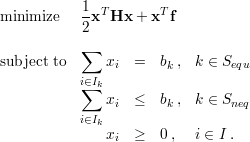whereis the optimized vector,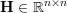is a symmetric positive semi-definite matrix,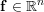is a vector,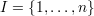is an index set,are subsets of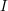such that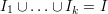and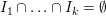,and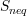are index sets such that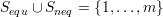and,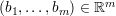are positive numbers.

The implemented solver (libqp_splx.c) is a generalization of the method proposed in [1, 2]. It is based on the Sequential Minimal Optimization (SMO) algorithm with an improved working set selection strategy. Solving instances of this QP task is required, for example, in machine learning methods like Structured SVM learning, Bundle Methods for Risk Minimization, binary SVM with L2-soft margin, etc.

QP task with box constraints and a single linear equality constraint. This QP task is defined as followswhere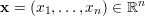is the optimized vector,is a symmetric positive semi-definite matrix,is a vector, a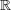n is a vector with non-zero entries, bis a scalar, (l1,,ln)(∪{-∞})n and (u1,,un)(∪{∞})n are lower and upper bounds, respectively.

The solver (libqp_gsmo.c) is the exact implementation of the Generalized Sequential Minimal Optimizer proposed in . Solving this QP task is required, for example, when training binary SVM with L1-soft margin.

### Interfaces

LIBQP is implemented in C language and interfaces to Matlab.

### Platforms

GNU/Linux. It should run also under Windows though not tested.

### Installation

MATLAB.

1. Run Matlab and go to the folder libqp_root/matlab
 cd libqp_root/matlab

2. Compile mex files by running
 libqp_compile

Now you can use libqp_splx and libqp_gsmo solvers located in libqp_root/matlab. To make these function visible from Matlab you need to add

To test the solvers run scripts

 libqp_splx_test    libqp_gsmo_test

Example application.

1. Go to the folder libqp_root/examples
 cd libqp_root/examples

2. Issue make
 make

Now you can run test script

 ./run_test